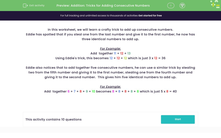### Comprehensive & curriculum aligned

In this worksheet, students learn a simple strategy for adding together 3 or more numbers.Key stage:  KS 2

Curriculum topic:   Number: Addition, Subtraction, Multiplication and Division

Curriculum subtopic:   Perform Mental Calculations

Popular topics:   Subtraction worksheets, Addition worksheets

Difficulty level:#### Worksheet Overview

In this worksheet, we will learn a crafty trick to add up consecutive numbers.

Eddie has spotted that if you steal one from the last number and give it to the first number, he now has three identical numbers to add up.

For Example:

Add  together 11 + 12 + 13

Using Eddie's trick, this becomes 12 + 12 + 12 which is just 3 x 12 = 36

Eddie also notices that to add together five consecutive numbers, he can use a similar trick by stealing two from the fifth number and giving it to the first number, stealing one from the fourth number and giving it to the second number.  This gives him five identical numbers to add up.

For Example:

Add  together 6 + 7 + 8 + 9 + 10 becomes 8 + 8 + 8 + 8 + 8 which is just 5 x 8 = 40

### What is EdPlace?

We're your National Curriculum aligned online education content provider helping each child succeed in English, maths and science from year 1 to GCSE. With an EdPlace account you’ll be able to track and measure progress, helping each child achieve their best. We build confidence and attainment by personalising each child’s learning at a level that suits them.

Get started